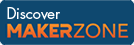Cody

# Problem 115. Distance walked 1D

Created by AMITAVA BISWAS in Community

Suppose you go from position 7 to 10 to 6 to 4. Then you have walked 9 units of distance, since 7 to 10 is 3 units, 10 to 6 is 4, and 6 to 4 is 2. Total distance traveled = 3 + 4 + 2 = 9.

Example:

``` Input  x = [1 2 3 2]
Output y is 3```

### Solution Stats

57.94% Correct | 42.06% Incorrect
Last solution submitted on Mar 18, 2019

#### TagsMATLAB and Simulink resources for Arduino, LEGO, and Raspberry Pi